# PI-Day

When using an arguable stupid American date format, today’s date looks like „03/14/15“ instead of 2015-03-14, which are the first few digits of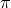.

Now we all know how to calculate, we just use the Taylor series ofor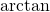, which unfortunately does not converge too well when used naïvely, but there are tricks to make it converge by calculating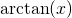or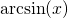of some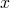with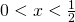resulting in a fraction ofthat can easily be multiplied to getfrom it. This approach is quite ok and understandable with relatively basic mathematical background, when allowing for some inaccuracy and intuition when trying to understand the Taylor theorem. But we can actually do better.is somehow weird for being so irrational and even non-algebraic and also in some other aspects, when investigating it.

So here are some cool approaches to calculatingthat are efficient and beatiful, but require a little bit more advanced math or trust to the ones who provided it (which should be ok for most people, but a no-go for mathematicians):

# AGM

Use the arithmetic-geometric mean, also mentioned shortly in Geometric and Harmonic Rounding:

The arithmetic-geometric mean is included in long-decimal, and long-decimal uses the AGM-method for calculating pi.

# Elliptic Integrals

Elliptic Integrals are somewhat weird, because they are easy to write down, but not really easy to solve. But numerical approximations to their calculation can also help finding about the value ofto some desired precision. Since elliptic functions, elliptic curves and elliptic integrals have fascinated mathematicians )and at least in the case of elliptic curves over finite fields also computer scientists) a lot, this must be cool.

# Other

If you just want to calculation 10000 digits on your computer (should be Linux or something with Ruby preinstalled), just type:
 gem install long-decimal ruby -e 'require "long-decimal";puts(LongMath.pi(10000))' 

That should give you some bed time lecture for the-day. Enjoy it.
And please, do not memorizeto 10000 digits. It is possible, but there is stuff more worth while memorizing.

And please, do use a decent date format, even if you are American.